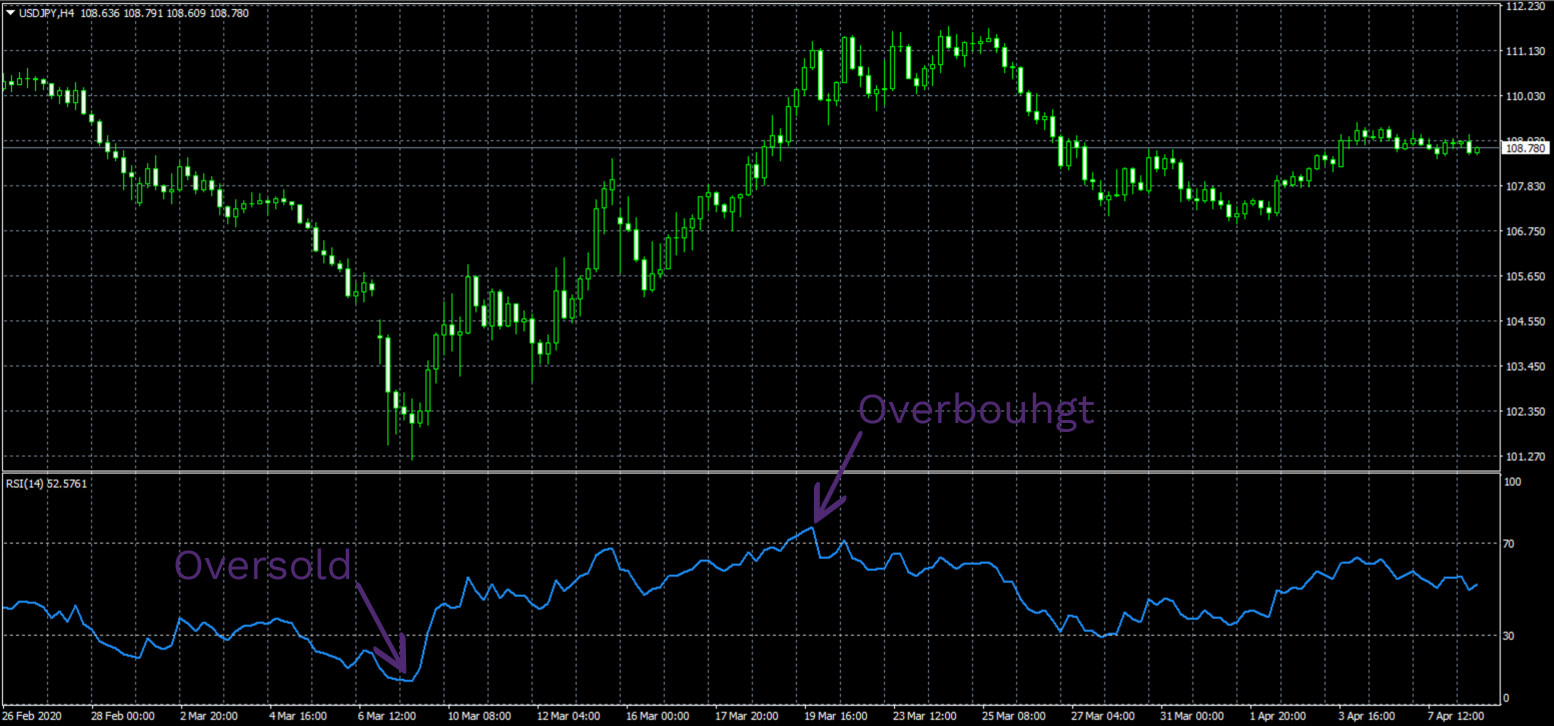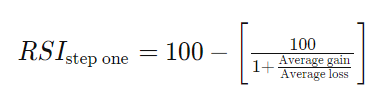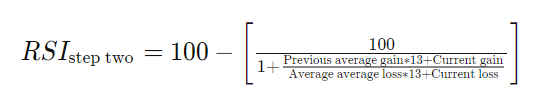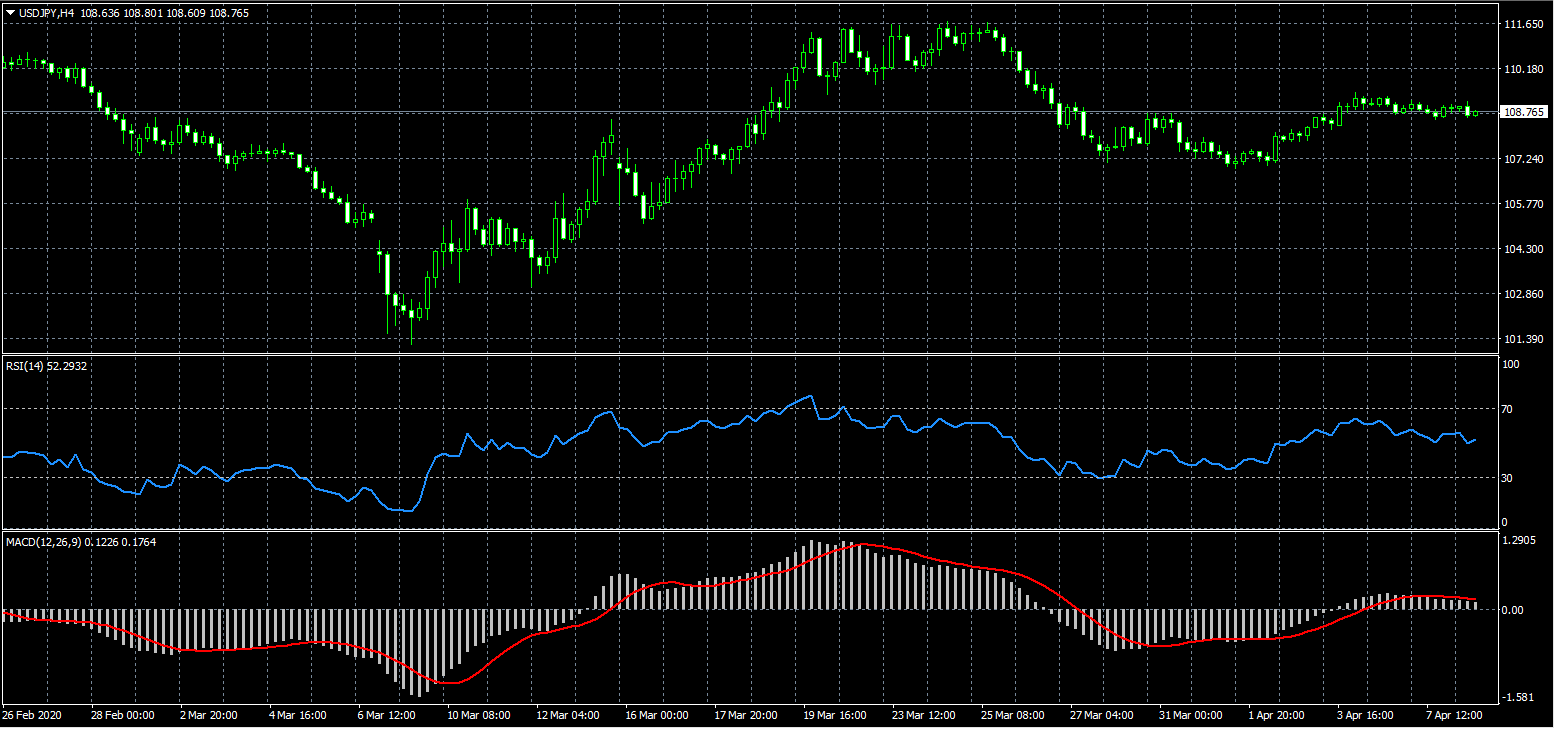• Accounts
• Platforms
• Education
• Partnerships
English

# How to Trade with the Relative Strength Index

Relative Strength Index, shortly known as the RSI, is a technical indicator that traders use to determine the momentum of a trend. It is shown as an oscillator - a line that moves from one extreme point to another and can indicate whether the market is overbought or oversold.

RSI uses a scale from 0 to 100 to rate the over-trending level of the market. Usually, traders get the overbought signal when the line graph goes above 70 on the scale. Conversely, if the line graph goes below 30, the indicator will show that the market is oversold.

All this in the Relative Strength Index chart means the following:

• If the market is overbought, there is a high chance that the uptrend will retrace and drop the price of an asset
• If the market is oversold, a trader may expect the downtrend to retrace and increase the price

To calculate the RSI score on a 0-100 scale, traders use the data of the past 14 days and a two-step formula, which we will explain in the article down below. Regardless of its intuitiveness, though, the RSI indicator has a few weaknesses as well. For example, a sharp increase/decrease in price can cause the indicator to give out false overbought/oversold signals.

## Start Trading in 10 Minutes

Apply everything you’ve learnt on a real trading account with up to 1:777 leverage, negative balance protection and outstanding support.

## Measuring the trend momentum - The RSI 101

One of the main characteristics of the trend, apart from its upward or downward direction, is its momentum - or the magnitude of the price changes that occurred recently. There are many technical indicators that help traders measure the momentum of the trend and the Relative Strength Indicator (RSI) is one of them.

By using the RSI indicator Forex traders can determine if the market is over-trending. It is an oscillator, which means the RSI line graph is constantly moving between the two extreme points from 0 to 100, and depending on the value of the RSI, the market will be overbought or oversold.

### Overbought and oversold markets

There are several ways of determining market momentum. The most popular one traders use is the RSI scores of 70 and 30:

• If the line graph goes above 70 in the chart, it means that the market is overbought
• If the line graph goes below 30, it shows that the market is oversoldAccording to the theory, when the market has been in an overbought/oversold condition for a long period of time, it tends to retract. Therefore, if we had an overbought market for a while, the price of an asset may start declining soon. Conversely, if the market has been oversold for a while, the price may start increasing.

There is another, more extreme scoring of the RSI indicator meaning the traders use not 70 and 30, but 80 and 20 as the extreme points. This method, according to those who use it, is more accurate for getting the signal and making more effective trading decisions.

### The formula for RSI

In order to calculate the actual value of the RSI graph, the indicator usually depends on the data from the previous 14 days of price movements. The formula consists of two parts, where the first part is calculated the following way:

RSI (step one) = 100 - [100/(1 + Average gain/Average loss)]In this formula, the average gain represents the average percentage gain of the asset price during the recent 14 days, while the average loss shows the average decline of the price in the same period. Now, while the average loss will be represented as a negative value, to calculate the RSI indicator Forex traders have to use the positive value of it. So, instead of, say, -1% change, the formula will use a 1% change.

The second part of the RSI formula looks like this:

RSI (step two) = 100 - [100/(1 + {previous average gain*13 + current gain}/{previous average loss*13 + current loss})]While the first part of the formula gives us a more sensitive RSI score, this second formula will make the results smoother, which means the score will only reach 0 or 100 values if the trend is in a strong upward or downward direction. Otherwise, small price increases and the number of days with such results will incrementally increase the RSI line, whereas the price declines, and the number of days with such results will slowly decrease the RSI line.

Once the actual RSI score is calculated, then traders can make a decision as to which trading position to take. The way they usually do that goes like this: if the RSI score gets closer or crosses above 70 on the scale, it means that the market has been overbought for the previous 14 days and it may be a good idea to sell an asset as its price may start declining.

In the opposite scenario, if the RSI score gets closer or crosses below 30, the indicator may give out the buy signal because the market has been in an oversold condition for a while and the price may start increasing soon.

To make the positions a bit safer, traders can use a stop-loss order just above the recent high - if they are selling an asset, or just below the recent low - if they are buying an asset. This way, the platform will automatically close the trade if it starts to generate losses.

### Some drawbacks of the Forex RSI explained

Now, while there are many advantages with the Relative Strength Index Forex traders can utilize, there are still some disadvantages that we need to discuss. For example, even though the second RSI formula we mentioned earlier smoothens the overall results, the drastic price changes can still cause the indicator to spike up or down, giving out false overbought or oversold signals.Another flaw of the RSI indicator is that the overbought/oversold conditions can last quite a while before the price starts to actually retract. For this reason, traders tend to use it alongside other indicators like MACD (Moving Average Convergence/Divergence). In a combination, the RSI will show whether the market is oversold/overbought while the MACD will detect the divergence from the current price, giving out the signal to buy or sell an asset.

## Start Trading in 10 Minutes

Apply everything you’ve learnt on a real trading account with up to 1:777 leverage, negative balance protection and outstanding support.

## The FX RSI guide - Key takeaways

In Forex, as well as other trading markets, traders can use many technical indicators to determine the momentum of the current trend. The Relative Strength Index (RSI) is one of those indicators widely used by many traders.

According to the FX RSI definition, the market, whether it is Forex, stocks, or anything else, tends to stay in an overbought/oversold condition for a while, which then leads to a drastic price contraction. For instance, if the market is overbought, the price will likely start declining soon.

To measure the over-trending condition of the market, the RSI uses a scale from 0 to 100. If the RSI line is near or crosses above 70, the market is overbought, whereas the RSI line at 30 shows an oversold market. For calculations, the indicator uses the data of the previous 14 days of price fluctuations.

In order to get more accurate signals from the RSI, traders tend to pair it with other indicators like MACD. This way, while the RSI shows the condition of the market - whether it’s overbought or oversold, the MACD will indicate the price divergence and give us an estimate for the next price retraction.

## FAQ on the RSI meaning in Forex

### 1.How can I use the RSI in Forex trading?

The Relative Strength Index (RSI) is used to measure the momentum or the strength of the asset price movements. It has a score from 0 to 100 that helps you determine the condition of the market - whether it’s overbought or oversold.

If the RSI Forex line is near 70 or is crossing it above, the market is overbought and there is a high chance that the price will start declining. Therefore, it may be a good idea to place a short position - sell your Forex pair. And to make it a little bit safer, you can set the stop-loss order at the recent high to prevent the position from going against you.

Conversely, if the RSI line is near or crossing below 30, it means the market is oversold and the price will likely start increasing. For this reason, you may want to place a long position - buy a Forex pair. The same stop-loss order can be set at the recent low to prevent the position to go even lower than that and lead you to a loss.

### 2.How can I locate and install the RSI indicator MT4 download file on my PC?

Usually, every MetaTrader 4 version comes with the Relative Strength Index already installed in it. But you may want to download another version of the indicator, which is actually pretty easy to do on a PC.

First things first, you need to find the actual file that you want to install. If you search it on Google, you will see lots of different sources that you can choose from. After you’ve found your best RSI version, you hit the download button and move on to the next step, which is to install it on MT4.

To do that, you need to take the downloaded file and put it in the MT4 Indicators folder. To locate it, go to the disk drive where you installed the platform, find the MetaTrader 4 folder, then open the MQL4 folder and drag the RSI trading file into the Indicators folder. The final step is to restart MT4 and find your new RSI indicator in it.

### 3.How to calculate the RSI score?

When using the RSI indicator MT4 will automatically calculate the trend momentum and give out respective signals for an overbought/oversold market. That said, there is actually a manual calculation formula for determining the actual value of the RSI line graph.

To calculate the RSI score, we need to take the data of the recent 14 days of price fluctuations. Then, we need to put those data in two consecutive formulas. The first one is as follows:

RSI (step one) = 100 - [100/(1 + Average gain/Average loss)]

In this formula, the average gain or loss is the average percentage gain or loss in the asset price during the recent 14 days. The second formula goes like this:

RSI (step two) = 100 - [100/(1 + {previous average gain*13 + current gain}/{previous average loss*13 + current loss})]

It helps to smooth out the graph and give more moderate signals.